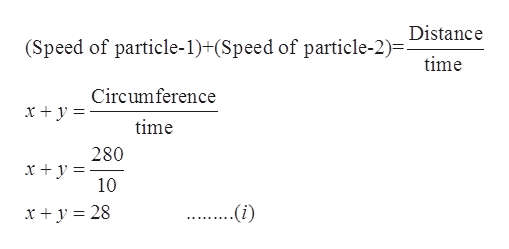# Two particles begin at the same point and move at different speeds along a circular path of circumference 280 ft. Moving in opposite directions, they pass in 10 sec. Moving in the same direction, they pass in 70 sec. Find the speed of each particle.

Question
2 views

Two particles begin at the same point and move at different speeds along a circular path of circumference 280 ft. Moving in opposite directions, they pass in 10 sec. Moving in the same direction, they pass in 70 sec. Find the speed of each particle.

check_circle

Step 1

Given: Two particles begin at the same point and move at different speeds along a circular path of circumference 280 ft. Moving in opposite directions, they pass in 10 sec. Moving in the same direction, they pass in 70 sec.

Step 2

Formula used:

Step 3

Let the speed of particle-1 is x and particle-2 is y and given the circumference of circular path is 280 ft. for moving ...help_outlineImage TranscriptioncloseDistance (Speed of particle-1)+(Speed of particle-2)=- time Circumference x +y = time 280 x + y = 10 ..(i) x + y = 28 fullscreen

### Want to see the full answer?

See Solution

#### Want to see this answer and more?

Solutions are written by subject experts who are available 24/7. Questions are typically answered within 1 hour.*

See Solution
*Response times may vary by subject and question.
Tagged in

### Equations and In-equations